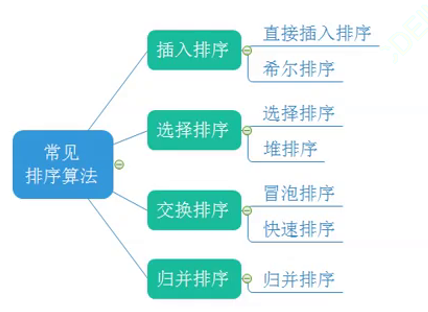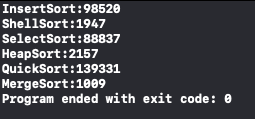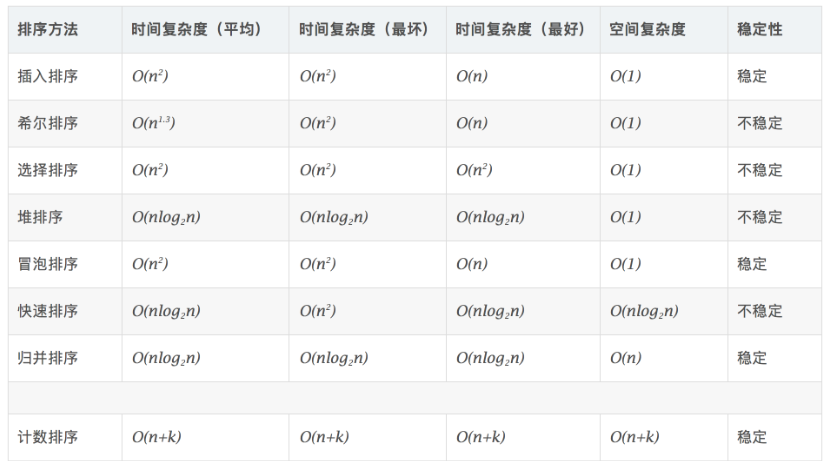WHCSRL 技术网

# 两万字搞定《数据结构》 八大排序 必读（建议收藏）## 1.插入排序## 1.1直接插入排序

``````//插入排序
void InsertSort(int* a, int n){
//控制起始条件
//注意控制好终止条件，这里的end的位置是在倒数第二个位置，所以要-1
for(int i=0; i<n-1;i++){
//单趟插入
int end = i;
int temp = a[end + 1]; //有序区间的后面
while(end>=0){ //end到-1就终止了
if(a[end]>temp){
a[end+1] = a[end];
--end;
}else{
break;
}
}
//两种情况：第一种在最右边，第二种在最左边，end为-1了，始终放在end后面
a[end+1] = temp;
}
}
```1234567891011121314151617181920```

## 1.2希尔排序gap越小，越接近有序，gap越大，越不接近有序；

``````void ShellSort1(int* a, int n)
{
int gap = n;

while (gap>1)//别傻乎乎的加等号啊，死循环
{
gap = gap / 3 + 1;end的范围是[0,n-gap)

for (int i = 0; i < n - gap; i++)//并排走
{
int end = i;
int temp = a[end + gap];
while (end>=0)
{
//当前的end的值比tmp大就要往end+gap位置挪
//所以要提前保存end+gap的值
if (temp < a[end])
{
a[end + gap] = a[end];
end = end-gap;
}
else
{
break;
}
}
a[end + gap] = temp;
}
}
}
```123456789101112131415161718192021222324252627282930```

O(N^1.3)，一般gap建议以gap/3+1的步骤走。

## 2.选择排序## 2.1 选择排序（二元改进版）

``````void SelectSort(int* a, int n)
{
assert(a);
int begin = 0, end = n - 1;
while (begin < end)
{
int min = begin, max = begin;
for (int i = begin; i <= end; i++)//注意起点是begin
{
if (a[i] >= a[max])
max = i;

if (a[i] < a[min])
min = i;
}
//最小的放在
Swap(&a[begin], &a[min]);
//如果最大的位置在begin位置
//说明min是和最大的交换位置
//这个时候max的位置就发生了变换
//max变到了min的位置
//所以要更新max的位置
if (begin == max)
max = min;

Swap(&a[end], &a[max]);
++begin;
--end;
}
}
```12345678910111213141516171819202122232425262728293031```

O(N^2)，最坏的排序

## 2.2 堆排序``````//堆排序
void AdjustDown(int* a, int n, int parent){
int child = parent*2 + 1;
while(child < n){
if(child+1<n && a[child+1] > a[child]){
++child;
}
if(a[child]>a[parent]){
Swap(&a[child], &a[parent]);
parent = child;
child = parent*2+1;
}else{
break;
}
}

}
void HeapSort(int* a, int n){
//排升序建大堆 O(N)
for(int i=(n-1-1)/2; i>=0; i--){
}

//O(N*logN)
int end = n - 1;
while(end > 0){
Swap(&a, &a[end]);
AdjustDown(a, end, 0); //是不是妙不可言hhh！
end--;
}
}
```12345678910111213141516171819202122232425262728293031```## 3.1 冒泡排序``````void BubbleSort(int* a, int n){

for(int j=0; j<n; j++){
int flag = 0;
for(int i=1; i<n-j; ++i){
if(a[i] < a[i-1] ){
Swap(&a[i], &a[i-1]);
flag = 1;
}
}
if(flag == 0){
break;
}
}
}
```123456789101112131415```

O(N^2)

## 3.2 快速排序

### 3.2.1 Hoare``````//三数取中
int MidIndex(int* a, int left, int right)
{
int mid = left + (right - left) / 2;
if (a[left] < a[mid])
{
if (a[mid] < a[right])
{
return mid;
}
else if (a[left] < a[right])
{
return right;
}
else
{
return left;
}
}
else //a[left] > a[mid]
{
if (a[mid] > a[right])
{
return mid;
}
else if (a[left] < a[right])
{
return left;
}
else
{
return right;
}
}
}
void Swap(int* a, int* b)
{
int tmp = *a;
*a = *b;
*b = tmp;
}
//不妨思考一下我们进行“三数取中”的意义是什么？

```12345678910111213141516171819202122232425262728293031323334353637383940414243```

``````
//一个单趟进行的排序操作的时间复杂度是多少？思考下一次完整的快排需要进行多少趟这样的单趟排序？

int PartSort1(int* a, int left, int right)
{
int midi = MidIndex(a, left, right);
Swap(&a[left], &a[midi]);

//最左边的做key为例
int key = left;
while (left<right)
{
//因为我们是最左边的取key，所以必须是右边先走找比key小的，思考下为什么？
//右边先走
while (left < right && a[right] >= a[key])
{
--right;
}
//然后左边走
while (left < right && a[left] < a[key])
{
++left;
}
Swap(&a[left], &a[right]);
}
Swap(&a[left], &a[key]);//此时left已经和right相遇，一样的
return left;
}
```12345678910111213141516171819202122232425262728```

``````void QuickSort(int* a, int left, int right)
{
//当区间分割到只剩一个或者没有的时候就返回
if (left >= right)
return;
//确定一个位置，划分区间递归
//分为[left,key-1]   key   [key+1，right]
//int key = PartSort1(a, left, right);
int key = PartSort2(a, left, right);
QuickSort(a, left, key - 1);
QuickSort(a, key + 1, right);
}
```123456789101112```

### 3.2.2 前后指针法

1.cur往前走，找到比key小的数据

2.找到比key小的数据以后，停下来，++prev

3.交换prev和cur指向位置的值

cur还没遇到比key大的数据之前，prev紧跟着cur，cur遇到比key大的值以后，prev和cur之间间隔着一段比key大的数据。

``````int PartSort2(int* a, int left, int right)
{
int midi = MidIndex(a, left, right);
Swap(&a[midi], &a[left]);

//这里key选取最左边的元素为例
int key = left;
int prev = left, cur = prev + 1;

while (cur<=right)
{
if (a[cur] < a[key] && ++prev != cur)//防止自己与自己交换
{
Swap(&a[cur], &a[prev]);
}
cur++;
}
//cur走到末尾啦，交换一下。
Swap(&a[prev], &a[key]);//这里可以保证交换之前a[prev]一定小于a[key],思考下为啥？
return prev;
}
```123456789101112131415161718192021```

``````void QuickSort(int* a, int left, int right)
{
//当区间分割到只剩一个或者没有的时候就返回
if (left >= right)
return;
//确定一个位置，划分区间递归
//分为[left,key-1]   key   [key+1，right]
//int key = PartSort1(a, left, right);
int key = PartSort2(a, left, right);
QuickSort(a, left, key - 1);
QuickSort(a, key + 1, right);
}
```123456789101112```

### 3.2.3 挖坑法``````int PartSort3(int* a, int left, int right)
{
int midi = MidIndex(a, left, right);
Swap(&a[midi], &a[left]);

//这里key取最左边的数，让右边的先开始走找小
int hole = left;
int key = a[left];

while (left < right)
{
//先找右边比key小的，填到左边的坑里面去
while (left < right && a[right] >= key)
{
right--;
}
a[hole] = a[right];
hole = right;

//再找左边比key大的，找到就交换坑位
while (left<right&&a[left]<key)
{
left++;
}
a[hole] = a[left];
hole = left;
}
a[left] = key;//最后把key放到相遇点
return left;
}
```123456789101112131415161718192021222324252627282930```

``````void QuickSort(int* a, int left, int right)
{
//当区间分割到只剩一个或者没有的时候就返回
if (left >= right)
return;
//确定一个位置，划分区间递归
//分为[left,key-1]   key   [key+1，right]
//int key = PartSort1(a, left, right);
int key = PartSort3(a, left, right);
QuickSort(a, left, key - 1);
QuickSort(a, key + 1, right);
}
```123456789101112```

## 3.3 快速排序（非递归）

1.当递归深度过大的时候，递归程序本身可能没用错误，但是编译之后会报错——栈溢出（stack overflow）。

2.性能问题（某些书上提到的，但是现在编译优化得很好，这个问题不大）。

1.循环(但是有的东西是不好改成循环的，比如二叉树的遍历、快排等)

2.“栈”模拟（这个“栈”是数据结构中的“栈”，不是系统内部那个“栈”，一般用到栈难度都是略大的）

``````void QuickSortNonR(int* a, int left, int right)
{
//非递归，我们可以处理当前的区间，再处理分区间
//先入右，后入左，就先拿到左
Stack s;
StackInit(&s);
StackPush(&s,right);
StackPush(&s,left);
while (!StackEmpty(&s))
{
left = StackTop(&s);
StackPop(&s);
right = StackTop(&s);
StackPop(&s);
//处理当前区间 [left,right]
int key = PartSort3(a, left, right);

//划分左右区间，分别入栈
//[left,key-1]    key    [key+1,right]
//先入右区间,区间有两个值才需要处理
if (key + 1 < right)
{
StackPush(&s, right);
StackPush(&s, key + 1);
}
//再入左区间
if (left < key - 1)
{
StackPush(&s, key - 1);
StackPush(&s, left);
}
}
}
```123456789101112131415161718192021222324252627282930313233```

## 4.归并排序## 4.1 递归实现归并排序

``````void _MergeSort(int* a, int left, int right,int* newArr)
{
if (left >= right)
return;
int mid = left + (right - left) / 2;
//[left,mid][mid+1,right]
_MergeSort(a, left, mid,newArr);
_MergeSort(a, mid + 1, right,newArr);
//走到这里已经是左右区间有序
//将两个区间合并成一个区间
//拷贝到newArr当中，排完再放回
int index = left;
int begin1 = left, end1 = mid;
int begin2 = mid+1,end2 = right;

while (begin1 <= end1 && begin2 <= end2)
{
if (a[begin1] < a[begin2])
{
newArr[index++] = a[begin1++];
}
else
{
newArr[index++] = a[begin2++];
}
}
//走到这里一定有一边没有走完
while (begin1 <= end1)
{
newArr[index++] = a[begin1++];
}
while (begin2 <= end2)
{
newArr[index++] = a[begin2++];
}
//拷贝回元素组  letf -- right 的位置
for (int i = left; i <= right; ++i)
{
a[i] = newArr[i];
}
}
void MergeSort(int* a, int n)
{
//归并排序就是在左右区间有序重新组合起来
//所以保证左右区间都是有序，遍历到叶子就可以
int* newArr = (int*)malloc(sizeof(int) * n);
int left = 0;
int right = n - 1;
_MergeSort(a, left, right,newArr);
}
```1234567891011121314151617181920212223242526272829303132333435363738394041424344454647484950```

O（NlogN)，可以看出他的递归过程中每次都将一组平均分，分完后高度大概是logN，空间复杂度O（N）

## 4.2 迭代实现归并排序

1.循环(但是有的东西是不好改成循环的，比如二叉树的遍历、快排等)

2.“栈”模拟（这个“栈”是数据结构中的“栈”，不是系统内部那个“栈”，一般用到栈难度都是略大的）

``````void MergeSortNonR(int* a, int n)
{
//int a[] = { 8,4,5,7,1,3,6,2,7,8 };

int* newArr=(int*)malloc(sizeof(int)*n) ;
int groupNum = 1;
int left;
int right;
//动态规划的思想，当我们把最小的问题切割
while(groupNum<n/2+1)
{
for (int i = 0; i < n; i += (2*groupNum))
{
//分成两组[begin1,end1][begin2,end2]
int begin1 = i;
int end1 = i + groupNum - 1;
int begin2 = i + groupNum;
int end2 = i + 2 * groupNum - 1;
//处理两种情况，当end1已经越界，说明处理end1的边界
if (end1 >= n)
{
end1 = n - 1;
}
//当end1越界，理所当然的begin2和end2都越界了
//这里可能的[begin1,end1]区间，也需要拷贝到临时数组，再拷回原数组
if (begin2 >= n)
{
//表示右区间不存在
begin2 = n;
end2 = n-1;
}
else if (begin2 < n && end2 >= n)
{
end2 = n - 1;
}

left = begin1;
right = end2;
//index用于放到临时数组newArr当中的
int index = begin1;

while (begin1 <= end1 && begin2 <= end2)
{
if (a[begin1] < a[begin2])
{
newArr[index++] = a[begin1++];
}
else
{
newArr[index++] = a[begin2++];
}
}
//走到这里一定有一边没有走完
while (begin1 <= end1)
{
newArr[index++] = a[begin1++];
}
while (begin2 <= end2)
{
newArr[index++] = a[begin2++];
}
//拷贝回元素组  letf -- right 的位置
for (int x = left; x <= right; ++x)
{
a[x] = newArr[x];
}
}
groupNum*=2;
}
free(newArr);
newArr = NULL;
}
```123456789101112131415161718192021222324252627282930313233343536373839404142434445464748495051525354555657585960616263646566676869707172```

O（NlogN)

## 5.计数排序1.统计原数组中每个值出现的次数

2.排序：遍历Count数组，对应位置的值出现多少次就往原数组写几个这个值

``````void CountSort(int* a, int n)
{
//int a[] = { 31,24,25,16,1,0,79 };

//遍历一遍找到最大和最小，然后开大一的数组
int max = a;
int min = a;
for (int i = 1; i < n; ++i)
{
if (max < a[i])
{
max = a[i];
}
if (min > a[i])
{
min = a[i];
}
}
int size = max - min + 1;
int* tmp = (int*)calloc(size,sizeof(int));
//将a遍历映射到tmp当中,a的长度是n，tmp的长度只有size
for (int i = 0; i < n; ++i)
{
tmp[a[i] - min]++;//tmp[i]存放的是这个值出现的次数
}
//在按照tmp当中的存放放回去
int index = 0;
for (int i = 0; i < size; ++i)
{
while (tmp[i] > 0)
{
//这里应该是下标+映射的
a[index++] = i + min;
--tmp[i];
}
}
}
```12345678910111213141516171819202122232425262728293031323334353637```

## 6.1八大排序的性能测试评估

``````// 测试排序的性能对比
void TestOP()
{
srand(time(0));
const int N = 10000;
int* a1 = (int*)malloc(sizeof(int)*N);
int* a2 = (int*)malloc(sizeof(int)*N);
int* a3 = (int*)malloc(sizeof(int)*N);
int* a4 = (int*)malloc(sizeof(int)*N);
int* a5 = (int*)malloc(sizeof(int)*N);
int* a6 = (int*)malloc(sizeof(int)*N);
int* a7 = (int*)malloc(sizeof(int)*N);

for (int i = 0; i < N; ++i)
{
a1[i] = rand();
a2[i] = a1[i];
a3[i] = a1[i];
a4[i] = a1[i];
a5[i] = a1[i];
a6[i] = a1[i];
a7[i] = a1[i];
}
int begin1 = clock();
InsertSort(a1, N);
int end1 = clock();

int begin2 = clock();
ShellSort(a2, N);
int end2 = clock();

int begin3 = clock();
SelectSort(a3, N);
int end3 = clock();

int begin4 = clock();
HeapSort(a4, N);
int end4 = clock();

int begin5 = clock();
QuickSort(a4, 0, N-1);
int end5 = clock();

int begin6 = clock();
MergeSort(a6, N);
int end6 = clock();
printf("InsertSort:%%%%%%%%%%%%%%%%%%%%%%%%%%%%%%%%d
", end1 - begin1);
printf("ShellSort:%%%%%%%%%%%%%%%%%%%%%%%%%%%%%%%%d
", end2 - begin2);
printf("SelectSort:%%%%%%%%%%%%%%%%%%%%%%%%%%%%%%%%d
", end3 - begin3);
printf("HeapSort:%%%%%%%%%%%%%%%%%%%%%%%%%%%%%%%%d
", end4 - begin4);
printf("QuickSort:%%%%%%%%%%%%%%%%%%%%%%%%%%%%%%%%d
", end5 - begin5);
printf("MergeSort:%%%%%%%%%%%%%%%%%%%%%%%%%%%%%%%%d
", end6 - begin6);
free(a1);
free(a2);
free(a3);
free(a4);
free(a5);
free(a6);
free(a7);
}
```123456789101112131415161718192021222324252627282930313233343536373839404142434445464748495051525354555657585960```## 6.3八大排序时间/空间复杂度一览